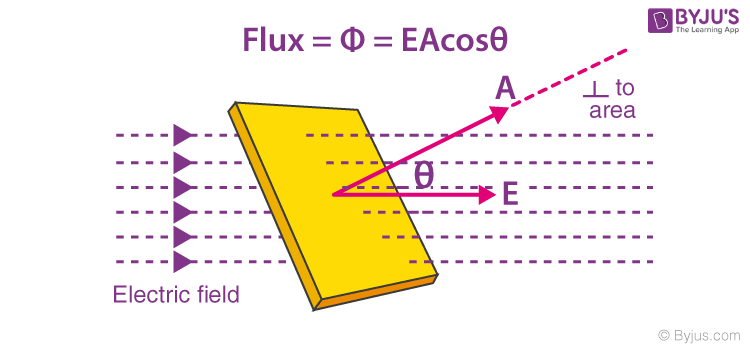# Electric Flux - Definition

## What is Electric Flux?

In this section, we will discuss the concept of electric flux, its calculation, and the analogy between the flux of an electric field and that of water. Let us imagine the flow of water with a velocity v in a pipe in a fixed direction, say to the right. If we take the cross-sectional plane of the pipe and consider a small unit area given by ds from that plane, the volumetric flow of the liquid crossing that plane normal to the flow can be given as vds. When the plane is not normal to the flow of the fluid but is inclined at an angle Ɵ, the total volume of liquid crossing the plane per unit time is given as vds.cosƟ. Here, dscosƟ is the projected area in the plane perpendicular to the flow of the liquid.

The electric field is analogous to the flow of liquid in the case shown above. The quantity we are going to deal with here is not an observable quantity as the liquid we considered above. Let us understand this with the help of the figure below.Here, we see that the electric field lines of magnitude E pass through a plane of area A that is kept at an angle Ɵ to the direction of the electric field.## Electric Flux Formula

The total number of electric field lines passing a given area in a unit time is defined as the electric flux. Similar to the example above, if the plane is normal to the flow of the electric field, the total flux is given as:

 $$\begin{array}{l}\phi _{p}=EA\end{array}$$

When the same plane is tilted at an angle Ɵ, the projected area is given as AcosƟ and the total flux through this surface is given as:

 $$\begin{array}{l}\phi=EAcos\Theta\end{array}$$

Where,

• E is the magnitude of the electric field
• A is the area of the surface through which the electric flux is to be calculated
• Ɵ is the angle made by the plane and the axis parallel to the direction of flow of the electric field

Watch this enticing video on Electric Flux and reimagine the concept like never before.See the video below to learn problems based on electric flux, electric charges and fields (Gauss’s law).Test Your Knowledge On Electric Flux!

1. What is an electric dipole?

1. Electric Dipole is a separation of positive and negative charges. The simplest example of this is a pair of electric charges of equal magnitude but opposite sign, separated by some distance.

2. Raja sohail

List the factors that affect the electric flux.

1. The electric flux through an area of element is given by the formula:
Φ=EAcosθ
From the formula, we see that electric flux depends on the following factors:

• Electric field through the area
• Surface area of the area element
• Angle of inclination between the field and normal to the area element
3. Shahzeb Daher

well explained